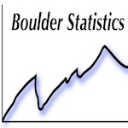Choose Language Hide Translation Bar
Highlighted

## Non-numeric Pass/Fail Rate Analysis

I'm a long time JMP user, but a first time poster.  I’ve searched and searched and cannot find a means to analyze data the way I’d really like.

Attached is an Example data table that I’m using.

For my specific example, each “ID” has ~700 unique X/Y coordinate locations.  And each one of those X/Y coordinate locations is tested for Pass/Fail.  (Pass = “.”; Fail = “F”).

Ideally, I’d like:

• A Contour Plot, with a color gradient associated to the Pass and/or Fail rate for all ID’s.  (e.g. if -9/+13 passes 1% of the time it would be blue, and if -9/+14 passes 99% of the time it would be red).
• Or a 3-dimensional chart indicating pass/fail rates similar to above.
• Or, if I can convert my X/Y coordinates into a distance-like measurement (radius, for example), a plot of Pass/Fail rate vs. distance.

The Example data table I have here (~30k rows) is a relative small subset of my larger data table (>>1 million rows).

Although I'm not opposed to working with scripts, can JMP do the above without scripts?  That is, does JMP have built-in functions to accomplish this task?

Help or advice is much appreciated.  Thanks.

3 REPLIES 3Super UserJoined:

Feb 10, 2013

## Re: Non-numeric Pass/Fail Rate Analysis

I think the key to your analysis is "data prep work".  Meaning that you if you had a table that had a %pass for each x-y coordinate then you could use any number of graphics to explore your data.  I would recode your pass/fail column to a numeric column with 0 = fail and 1 = pass.  Then you can summarize your table (your x and y go into the "Group" and then you want the mean for the P/F column.  Once you have that table try graph builder with x = x, y = y and the mean column (%pass) as the color and try the contour or heat map from there.

Karen

## Re: Non-numeric Pass/Fail Rate Analysis

Thank you very much.  This works beautifully.

## Re: Non-numeric Pass/Fail Rate Analysis

JMP appears to automatically interpret the Pass/Fail as a binary variable and show the proportion of "F" for each coordinate without summarizing. Recoding 0/1 gives the same result.

Set X and Y to Ordinal data type to get a heat map as below.